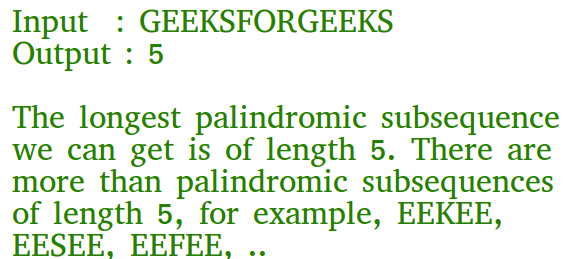Python Program for Longest Palindromic Subsequence | DP-12

• Last Updated : 17 Jan, 2022

Given a sequence, find the length of the longest palindromic subsequence in it.As another example, if the given sequence is “BBABCBCAB”, then the output should be 7 as “BABCBAB” is the longest palindromic subseuqnce in it. “BBBBB” and “BBCBB” are also palindromic subsequences of the given sequence, but not the longest ones.
1) Optimal Substructure:
Let X[0..n-1] be the input sequence of length n and L(0, n-1) be the length of the longest palindromic subsequence of X[0..n-1].
If last and first characters of X are same, then L(0, n-1) = L(1, n-2) + 2.
Else L(0, n-1) = MAX (L(1, n-1), L(0, n-2)).
Following is a general recursive solution with all cases handled.
Dynamic Programming Solution

Python3

 # A Dynamic Programming based Python# program for LPS problem Returns the length# of the longest palindromic subsequence in seqdef lps(str):    n = len(str)     # Create a table to store results of subproblems    L = [[0 for x in range(n)] for x in range(n)]     # Strings of length 1 are palindrome of length 1    for i in range(n):        L[i][i] = 1     # Build the table. Note that the lower    # diagonal values of table are    # useless and not filled in the process.    # The values are filled in a    # manner similar to Matrix Chain    # Multiplication DP solution (See    # dynamic-programming-set-8-matrix-chain-multiplication/    # cl is length of substring    for cl in range(2, n + 1):        for i in range(n-cl + 1):            j = i + cl-1            if str[i] == str[j] and cl == 2:                L[i][j] = 2            elif str[i] == str[j]:                L[i][j] = L[i + 1][j-1] + 2            else:                L[i][j] = max(L[i][j-1], L[i + 1][j]);     return L[n-1] # Driver program to test above functionsseq = "GEEKS FOR GEEKS"n = len(seq)print("The length of the LPS is " + str(lps(seq))) # This code is contributed by Bhavya Jain
Output:
The length of the LPS is 7

Time Complexity :- O(n2)

Please refer complete article on Longest Palindromic Subsequence | DP-12 for more details!

My Personal Notes arrow_drop_up